## Year 5 maths worksheet: multiplication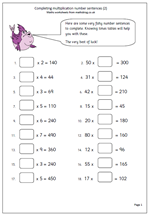Here is the second in a mini-series of maths worksheets that look at using knowledge of multiplication and tables to complete number sentences.

Again, the most important aspect of this page is to illicit what processes children use to answer the questions, and it is a worthwhile experience to ask yourself exactly how you went about it in your mind to reach the answer – it is not necessarily the way we would explain to children, yet it could well be a very effective way. Let’s look at a couple of the questions;

Question 3: ? x 4 = 44.

This I can answer without doing any kind of calculation in my head because I know, off by heart, that 4 x 11 = 44.

Question 7: ? x 5 = 110

A harder question which can be tackled several ways. I actually worked from knowing that 20 fives make 100 and then adding two more fives for the ten, making the answer 22. I probably wouldn’t suggest this to children. What I would suggest is double the 110 to make 220 and then divide by ten to make 22 (and explaining why this method works).

Complete multiplication number sentences (2)

## Complete multiplication number sentences (3)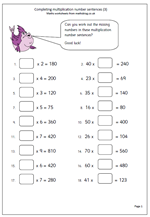This is the third worksheet in the mini series looking at using different strategies to work out missing numbers in multiplication number sentences. Again, it is worth pointing out that people who say they, ‘can’t do maths’, probably don’t have a wide range of mental strategies to tackle questions. All the questions on this worksheet look very similar but if you analyse how the brain works to answer them you will see what I mean.

Let’s look at a couple of examples:

1. Question: ? x 6 = 360

I instantly recognise the relationship between 6 and 36, knowing that 6 x 6 = 36. it then becomes very easy to multiply by 10 to reach the answer 60. This is all done in less than a second.

2. Question: 26 x ? = 104

This takes a little longer and at first glance I’m not certain. I then realised that 25 is a quarter of 100, so 25 x 4 is 100,therefore 26 x 4 would be 104.

In the first question I am making use of knowing the 6x table. In the second question I am rounding a number, working out the answer and adjusting.

Of course, there are other ways, probably just as good  and don’t forget to ask the children how they worked out the answers. You might be surprised!

Complete multiplication number sentences (3)

## Year 6 maths worksheet: ordering decimals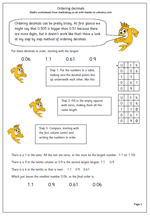Ordering decimals can be pretty tricky. At first glance we might say that 0.509 is bigger than 0.51 because there are more digits, but it doesn’t work like that! Have a look at my step by step method of ordering decimals.

Step 1: Put the numbers in a table, making sure the decimal points line up underneath each other.

Step 2: Fill in the empty squares with zeros, making them all the same length.

Step 3: Compare, starting with the first column (units) and writing the numbers in order.

This is quite a nifty way of making sure that you have got the numbers in order, although it is a little time consuming. Grids have been provided on the worksheets, but if a question like this comes up in the SAT tests it should be sufficient to just write the numbers in columns, not forgetting to put them in the answer page at the end!

Order decimals

## Year 4 maths worksheet: multiplying by 5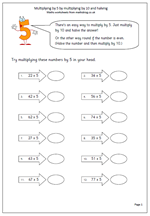By the end of year 4 children should be developing a wide range of strategies for working answers out ‘in their heads’. To be successful they do need plenty of practice with these strategies. One such technique is to be able to efficiently multiply 2-digit numbers by 5. Sometimes the best way to do this is to take the tens digit and multiply by 5 then do the same with the units. On other occasions it is easier to multiply the whole number by ten and then halve the answer; or, if the number is even, halve the number and multiply by ten.

This worksheet looks more closely at this .it is not always the best option, but it is ceertainly one that children should be confident with using.

Multiply by 5

## Multiplying 2 and 3-digit numbers by 10 or 100Today I have published another multiplying worksheet, suitable for Year 4 children. Of course, multiplying by ten is easy; just move each digit one place to the left and place a zero in the units. Repeat this for multiplying by 100, but avoid the trap of saying ‘add a nought’.

This page has incomplete number sentences. Often the answer is given and the numbers to be multiplied have to be found. This leads to a little more thought and develops the relationship between multiplication and division.

This page can be found in the Year 4 Calculating section of the site.

Multiplying 2 or 3-digit numbers by 10 or 100

## Completing multiplication number sentences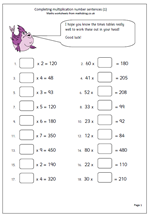This maths worksheet is suitable for Year 5 children who have a good knowledge of the times tables and can manipulate numbers ‘in their heads’.

It is surprising the number of different strategies we use to calculate mentally. The same type of question can be processed several different ways, often depending on the numbers being use. If we look at some of the questions on this worksheet it will become clearer as to what I mean, as I try to explain how I go about answering them, although you may well have different (and better) alternatives.

Question 1: ? x 2 = 120

Looking at the question I immediately think that I have to halve 120 to get the answer. I do this by halving 12, which is 6 and multiplying by 10, making 60. This is all done in a split second, and I might be tempted to think I did it in one, but it is important to stop and think of the steps that you go through.

Question 3: ? x 4 = 48

I could have halved and halved, or divided by 4, but, in fact, I learned my 12 times table many years ago and I know, instantly,  that 12 x 4 = 48 so the answer of 12 came immediately.

Question 4: 41 x ? = 205

The answer was not immediately obvious. I looked at the unit (1) and the unit in the answer (5) and it struck me that I need to multiply by 5. A quick check that4 x 5 will give me 20 confirmed this.

These are just a few of the ways of working out the answers and it is well worth asking children how they go about finding the answers.

Complete multiplication number sentences (1)

## Long multiplication worksheets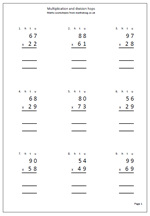Here is a page of practice questions for long multiplication using the standard written method. Each question is laid out so that the answer can be completed in three parts.

Looking at 67 x 23, the three stages would be:

1. multiply 67 by 20. The easiest way to do this is to place a zero in the units column of the answer and then multiply by 2. Placing the zero has the effect of moving each digit one place to the left, hence ten times bigger.

2. multiply by the units (3) and place the answer below, making sure the units go in the units column etc.

Of course the units could be multiplied first, followed by the tens, but it is important to find one standard method and stick to it. Of course, tables to to be known to make this a quick process.

This worksheet, and others, similar can be found in the Four Rules section of the site, under Multiplication.

Long multiplication 2-digits by 2-digits

## Multiply the Egyptian way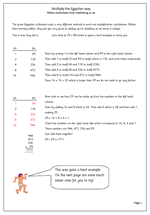Sometimes it can help children’s understanding of maths and numbers if they are shown a completely different way of approaching a calculation. In Year 6 most children will be familiar with the standard written method of multiplication, but this method is far from the only one which can be used. It just happens to be quite an efficient method which is relatively easy to use.

The great Egyptian civilisation used a very different method to work out multiplication calculations. Rather than learning tables, they just got very good at adding up pairs of the same number (or doubling as we know it today). One of the reasons for this is that they did not have multiplication ‘tables’.

The method is quite long, but nevertheless quite easy if you are good at addition and it is explained in detail on the first worksheet.

Egyptian multiplication

## Long multiplication: 3-digits by 2-digitsA standard page of long multiplication questions here. For children to be successful with these they need to have a good knowledge of ‘times tables’. Without this knowledge the whole process becomes quite tedious and errors can slip in even if the method is correct.

The standard method of long multiplication is one which most adults will be familiar with. It does not really matter if the number is multiplied by the tens first or the units first, as long as it is remembered to place a zero in the units column when multiplying by the tens digit. It is also important to line up the answer so that hundreds, tens and units are directly under each other: adding the final total can be very difficult if this is not done.

Long multiplication 3 digits by 2 digits

## Resource of the Week: Written multiplication of moneyMultiplication of money by a single digit is very much like multiplying a 3-digit number by a single digit, but, of course, the decimal point needs to be included. It would normally be expected to answer these types of question using the standard written method.

The first 8 questions are set out in the standard way but it is important that the second set of questions, from 9 to 15 should be set out in the correct way and not attempted as shown.

When marking these check that the working is shown, especially that the numbers have been carried across. It is often a good idea for children to check the answers to this type of question by using a calculator. (If they just use the calculator to find the answer this is quite obvious as there is no working shown!)

This page any many others can be found in our Four Rules section.

Multiplication of money (pg 1)